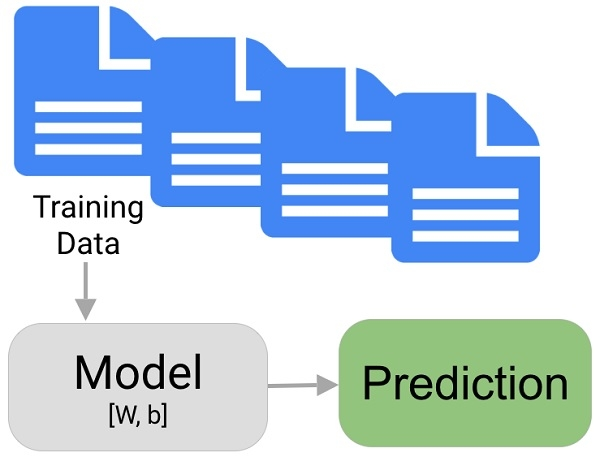# Introduction To Machine Learning using Python

In this article, we will learn about the basics of machine learning using Python 3.x. Or earlier.

First, we need to use existing libraries to set up a machine learning environment

>>> pip install numpy
>>> pip install scipy
>>> pip install matplotlib
>>> pip install scikit-learn

Machine learning deals with the study of experiences and facts and prediction is given on the bases of intents provided. The larger the database the better the machine learning model is.

The flow of Machine Learning

• Cleaning the data
• Feeding the dataset
• Training the model
• Testing the dataset
• Implementing the modelNow let’s identify which library is used for what purpose −

Numpy − adds support for huge, multi-dimensional lists and matrices, along with a wide collection of mathematical functions to operate on these input arrays.

SciPy − a free and open-source Python library which is used for scientific/mathematical computing. It contains modules for optimization of the algorithm, integration of data, interpolation, some special functions & linear algebra

Matplotlib − A library used for the formation of charts and figures. It allows plotting the data so as to gain a better insight into the model

Scikit-learn − it has the various classification, clustering and regression algorithms to distribute and organise the data in a well-defined manner

Now let’s make a basic machine learning model by the help of scikit - learn. Here we will take inbuilt datasets i.e. the iris & digits datasets available in ski-kit learn.

from sklearn import datasets
digits = datasets.load_digits()

Now to see the data from the datasets we use

print(digits.data)

[[ 0. 0. 5. ... 0. 0. 0.]
[ 0. 0. 0. ... 10. 0. 0.]
[ 0. 0. 0. ... 16. 9. 0.]
...
[ 0. 0. 1. ... 6. 0. 0.]
[ 0. 0. 2. ... 12. 0. 0.]
[ 0. 0. 10. ... 12. 1. 0.]]

.target function allows us to see the things we want our model to learn

digits.target

array([0, 1, 2, ..., 8, 9, 8])

for accessing the shape of the digits datasets we use

digits.images

array([[ 0., 0., 5., 13., 9., 1., 0., 0.],
[ 0., 0., 13., 15., 10., 15., 5., 0.],
[ 0., 3., 15., 2., 0., 11., 8., 0.],
[ 0., 4., 12., 0., 0., 8., 8., 0.],
[ 0., 5., 8., 0., 0., 9., 8., 0.],
[ 0., 4., 11., 0., 1., 12., 7., 0.],
[ 0., 2., 14., 5., 10., 12., 0., 0.],
[ 0., 0., 6., 13., 10., 0., 0., 0.]])

Now let's move to the learning and prediction part

from sklearn import svm
clf = svm.SVC(gamma=0.001, C=100.)

Here SVC is support vector classification which acts as an inbuilt estimator for our model

clf.fit(digits.data[:-1], digits.target[:-1])
SVC(C=100.0, cache_size=200, class_weight=None, coef0=0.0, decision_function_shape='ovr', degree=3, gamma=0.001,
kernel='rbf', max_iter=-1, probability=False, random_state=None,
shrinking=True, tol=0.001, verbose=False)

First we need to feed the model with dataset by using fit method so that our model can learn Here we feed all images as training data except the last image that we will use for testing purpose.

Now as our model is trained we can predict the output of the testing data by using .predict function

clf.predict(digits.data[-1:])
array()

Now as our model is trained we can compute the efficiency and time-cycle of our model

## Conclusion

In this article, we learnt about some basics of machine learning and some basic libraries used to implement it in Python.

Updated on: 28-Aug-2019

137 Views